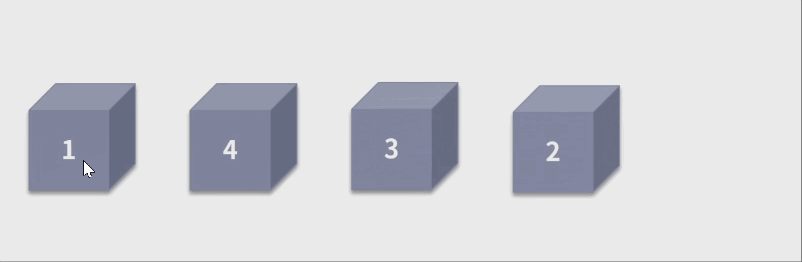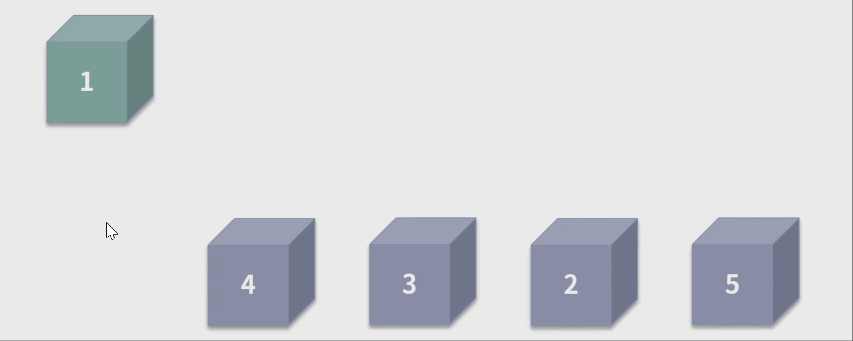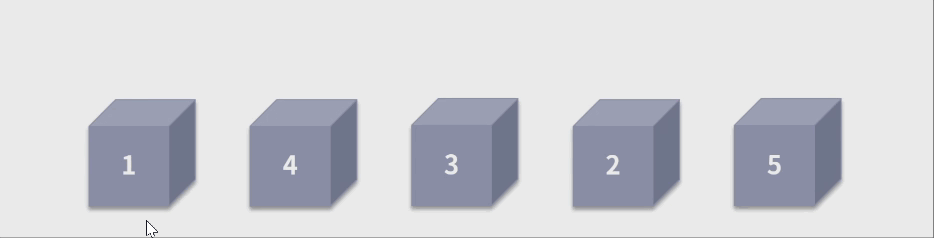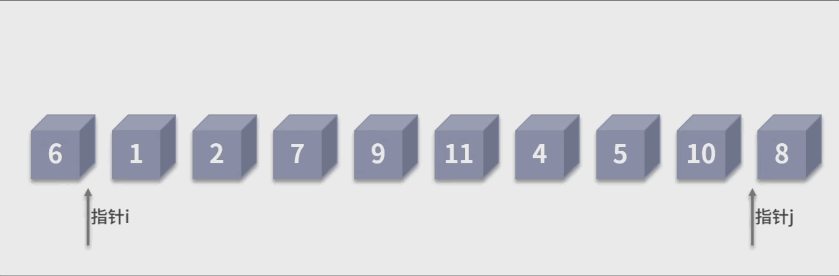# 常见的4种排序方法（冒泡排序、插入排序、归并排序、快速排序）

#### 1. 什么是排序问题

1. 时间复杂度，具体包括，最好时间复杂度、最坏时间复杂度以及平均时间复杂度。
2. 空间复杂度，如果空间复杂度为 1，也叫作原地排序。
3. 稳定性，排序的稳定性是指相等的数据对象，在排序之后，顺序是否能保证不变。

#### 2. 常见的4种排序算法及其思想

##### 2.1 冒泡排序

2.1.1冒泡排序的原理2.1.2冒泡排序的性能

1. 时间复杂度：最好时间复杂度是 O(n)；最坏时间复杂度是 O(n*n)；平均时间复杂度是 O(n *n)。
2. 空间复杂度： O(1)
3. 稳定性：稳定

public static void main(String[] args) {
int[] arr = { 1, 0, 3, 4, 5, -6, 7, 8, 9, 10 };
System.out.println("原始数据: " + Arrays.toString(arr));
for (int i = 1; i < arr.length; i++) {
for (int j = 0; j < arr.length - i; j++) {
if (arr[j] > arr[j + 1]) {
int temp = arr[j];
arr[j] = arr[j + 1];
arr[j + 1] = temp;
}
}
}
System.out.println("冒泡排序: " + Arrays.toString(arr));
}

##### 2.2 插入排序

2.2.1 插入排序的原理2.2.2 插入排序的性能

• 时间复杂度：最好时间复杂度是 O(n)；最坏时间复杂度是 O(n*n)；平均时间复杂度是 O(n *n)。
• 空间复杂度： O(1)
• 稳定性：稳定
代码如下：
public static void main(String[] args) {
int[] arr = { 2, 3, 5, 1, 23, 6, 78, 34 };
System.out.println("原始数据: " + Arrays.toString(arr));
for (int i = 1; i < arr.length; i++) {
int temp = arr[i];
int j = i - 1;
for (; j >= 0; j--) {
if (arr[j] > temp) {
arr[j + 1] = arr[j];
} else {
break;
}
}
arr[j + 1] = temp;
}
System.out.println("插入排序: " + Arrays.toString(arr));
}


• 相同点
插入排序和冒泡排序的平均时间复杂度都是 O(n*n)，且都是稳定的排序算法，都属于原地排序。
• 差异点
冒泡排序每轮的交换操作是动态的，所以需要三个赋值操作才能完成；
而插入排序每轮的交换动作会固定待插入的数据，因此只需要一步赋值操作。
##### 2.3 归并排序

1、归并排序的原理public static void main(String[] args) {
int[] arr = { 49, 38, 65, 97, 76, 13, 27, 50 };
int[] tmp = new int[arr.length];
System.out.println("原始数据: " + Arrays.toString(arr));
customMergeSort(arr, tmp, 0, arr.length - 1);
System.out.println("归并排序: " + Arrays.toString(arr));
}

public static void customMergeSort(int[] a, int[] tmp, int start, int end) {
if (start < end) {
int mid = (start + end) / 2;
// 对左侧子序列进行递归排序
customMergeSort(a, tmp, start, mid);
// 对右侧子序列进行递归排序
customMergeSort(a, tmp,mid + 1, end);
// 合并
customDoubleMerge(a, tmp, start, mid, end);
}
}

public static void customDoubleMerge(int[] a, int[] tmp, int left, int mid, int right) {
int p1 = left, p2 = mid + 1, k = left;
while (p1 <= mid && p2 <= right) {
if (a[p1] <= a[p2])
tmp[k++] = a[p1++];
else
tmp[k++] = a[p2++];
}
while (p1 <= mid)
tmp[k++] = a[p1++];
while (p2 <= right)
tmp[k++] = a[p2++];
// 复制回原素组
for (int i = left; i <= right; i++)
a[i] = tmp[i];
public static void main(String[] args) {
int[] arr = { 49, 38, 65, 97, 76, 13, 27, 50 };
int[] tmp = new int[arr.length];
System.out.println("原始数据: " + Arrays.toString(arr));
customMergeSort(arr, tmp, 0, arr.length - 1);
System.out.println("归并排序: " + Arrays.toString(arr));
}

public static void customMergeSort(int[] a, int[] tmp, int start, int end) {
if (start < end) {
int mid = (start + end) / 2;
// 对左侧子序列进行递归排序
customMergeSort(a, tmp, start, mid);
// 对右侧子序列进行递归排序
customMergeSort(a, tmp,mid + 1, end);
// 合并
customDoubleMerge(a, tmp, start, mid, end);
}
}

public static void customDoubleMerge(int[] a, int[] tmp, int left, int mid, int right) {
int p1 = left, p2 = mid + 1, k = left;
while (p1 <= mid && p2 <= right) {
if (a[p1] <= a[p2])
tmp[k++] = a[p1++];
else
tmp[k++] = a[p2++];
}
while (p1 <= mid)
tmp[k++] = a[p1++];
while (p2 <= right)
tmp[k++] = a[p2++];
// 复制回原素组
for (int i = left; i <= right; i++)
a[i] = tmp[i];



2、归并排序的性能

• 时间复杂度：
它采用了二分的迭代方式，复杂度是 logn。每次的迭代，需要对两个有序数组进行合并，这样的动作在 O(n) 的时间复杂度下就可以完成。因此，**归并排序的复杂度就是二者的乘积 O(nlogn)。**同时，它的执行频次与输入序列无关，因此，归并排序最好、最坏、平均时间复杂度都是 O(nlogn)。
• 空间复杂度： O(n)
• 稳定性：稳定
##### 2.4 快速排序

1、快速排序法的原理public static void main(String[] args) {
int[] arr = { 6, 1, 2, 7, 9, 11, 4, 5, 10, 8 };
System.out.println("原始数据: " + Arrays.toString(arr));
customQuickSort(arr, 0, arr.length - 1);
System.out.println("快速排序: " + Arrays.toString(arr));
}

public void customQuickSort(int[] arr, int low, int high) {
int i, j, temp, t;
if (low >= high) {
return;
}

i = low;
j = high;
temp = arr[low];
while (i < j) {
// 先看右边，依次往左递减
while (temp <= arr[j] && i < j) {
j--;
}
// 再看左边，依次往右递增
while (temp >= arr[i] && i < j) {
i++;
}
t = arr[j];
arr[j] = arr[i];
arr[i] = t;
}
arr[low] = arr[i];
arr[i] = temp;
// 递归调用左半数组
customQuickSort(arr, low, j - 1);
// 递归调用右半数组
customQuickSort(arr, j + 1, high);
}


2、快速排序法的性能

• 时间复杂度：最好时间的复杂度O(n*logn)；最坏的时间复杂度O(n *n) ；快速排序法在大部分情况下，统计上是很难选到极端情况的。因此它平均的时间复杂度是 O(n *logn)。
• 空间复杂度： O(1)
• 稳定性：不稳定

#### 3. 总结

4 种常见的排序算法，包括冒泡排序、插入排序、归并排序以及快速排序。这些经典算法没有绝对的好和坏，它们各有利弊。在工作过程中，需要你根据实际问题的情况来选择最优的排序算法。

• 归并排序的空间复杂度为 O(n)，也就意味着当排序 100M 的数据，就需要 200M 的空间，所以对空间资源消耗会很多。
• 快速排序在平均时间复杂度为 O(nlogn)，但是如果分区点选择不好的话，最坏的时间复杂度也有可能逼近
O(n*n)。而且快速排序不具备稳定性，这也需要看你所面对的问题是否有稳定性的需求。
09-1108-311738
05-09279
04-301122
01-161398
08-17944
07-101813
04-013763